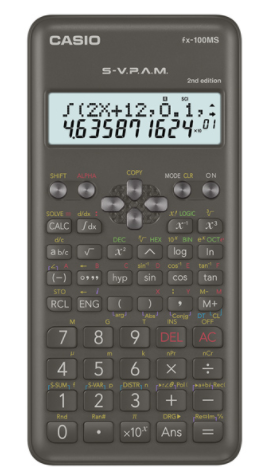# Casio FX-100MS-2 2nd Edition Scientific Calculator #C76B*Image may differ with actual product's layout, color, size & dimension. No claim will be accepted for image mismatch.

# Casio FX-100MS-2 2nd Edition Scientific Calculator #C76B

Product Id: 130.01.685.44

Regular Price  1,180
Special Price  1,100

Availability?

Quick Overview

Model - Casio FX-100MS-2 2nd Edition
Brand - Casio
Type - Scientific Calculator
Display - Dot matrix display
Digit - 12 digits (10 + 2 digits)
Key - Plastic keys
Model
Casio FX-100MS-2 2nd Edition
Type
Scientific Calculator
Display
Dot Matrix Display
Memory
No
Digit
12 (10 + 2 Digits)
Key
Plastic keys
Number of Functions
300
TAX Calculation
No
Power Sources
1 x AAA Battery
Dimensions
161.5 x 77 x 13.8mm
Weight (Kg)
105gm
Specialty
2nd Edition Scientific Calculator, Fraction calculations, Combination and permutation, Statistics (STAT-data editor, Standard deviation, Regression analysis), 9 variable memories, Comes with slide-on hard case, 10 + 2 digits, Dot matrix display, Multi-replay, Plastic keys, S-V.P.A.M. (Super Visually Perfect Algebraic Method), STAT-data editor, Non Graphing, Differentiation, AAA-size Battery, Equation, Basic Mathematical Functions, Complex number calculation, Base-n calculation, Integration, Basic Statistics
Others
Part No: C76B, 10 + 2 digits: 10-digit mantissa + 2-digit exponential display, Dot matrix display: High-resolution screen provides beautiful looking graphs every time, Multi-replay: Quick and easy recall of previously executed formulas for editing and re-execution, Plastic keys: Designed and engineered for easy operation, S-V.P.A.M. (Super Visually Perfect Algebraic Method): All the features of the existing V.P.A.M. series plus a new 2-line display and a useful Replay function, STAT-data editor: Back-step viewing and editing of input data, Basic Mathematical Functions: Trigonometric, Exponential logarithmic, etc., Base-n calculation: Binary/Octal/Hexadecimal, Basic Statistics: Standard statistics functions such as Mean, SUM, Standard Deviation, and Regression
Warranty
3 year
China
Country Of Origin
Japan

## fx-100MS-2## Features

• Fraction calculations
• Combination and permutation
• Statistics (STAT-data editor, Standard deviation, Regression analysis)
• 9 variable memories
• Comes with slide-on hard case

### fx-82MS/85MS/350MS functions, in addition to:

• Equation calculations
• Integration/differential calculations
• Base-n calculations/conversions
• Complex number calculations

Brand - Casio, Model - Casio FX-100MS-2 2nd Edition, Type - Scientific Calculator, Digit - 12 (10 + 2 digits), Display - Dot matrix display, Key - Plastic keys, Number of Functions - 300, TAX Calculation - No, Memory - No, Power source - 1 x AAA Battery, Dimension - 161.5 x 77 x 13.8mm, Weight - 105gm, Others - Part No: C76B, 10 + 2 digits: 10-digit mantissa + 2-digit exponential display, Dot matrix display: High-resolution screen provides beautiful looking graphs every time, Multi-replay: Quick and easy recall of previously executed formulas for editing and re-execution, Plastic keys: Designed and engineered for easy operation, S-V.P.A.M. (Super Visually Perfect Algebraic Method): All the features of the existing V.P.A.M. series plus a new 2-line display and a useful Replay function, STAT-data editor: Back-step viewing and editing of input data, Basic Mathematical Functions: Trigonometric, Exponential logarithmic, etc., Base-n calculation: Binary/Octal/Hexadecimal, Basic Statistics: Standard statistics functions such as Mean, SUM, Standard Deviation, and Regression, Specialty - 2nd Edition Scientific Calculator, Fraction calculations, Combination and permutation, Statistics (STAT-data editor, Standard deviation, Regression analysis), 9 variable memories, Comes with slide-on hard case, 10 + 2 digits, Dot matrix display, Multi-replay, Plastic keys, S-V.P.A.M. (Super Visually Perfect Algebraic Method), STAT-data editor, Non Graphing, Differentiation, AAA-size Battery, Equation, Basic Mathematical Functions, Complex number calculation, Base-n calculation, Integration, Basic Statistics, Warranty - 3 year, Country of Origin - Japan